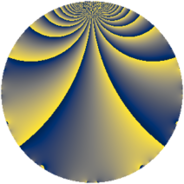# Properties

 Label 1205.2.bhLevel $1205$ Weight $2$ Character orbit 1205.bh Rep. character $\chi_{1205}(6,\cdot)$ Character field $\Q(\zeta_{20})$ Dimension $656$ Sturm bound $242$

# Related objects

## Defining parameters

 Level: $$N$$ $$=$$ $$1205 = 5 \cdot 241$$ Weight: $$k$$ $$=$$ $$2$$ Character orbit: $$[\chi]$$ $$=$$ 1205.bh (of order $$20$$ and degree $$8$$) Character conductor: $$\operatorname{cond}(\chi)$$ $$=$$ $$241$$ Character field: $$\Q(\zeta_{20})$$ Sturm bound: $$242$$

## Dimensions

The following table gives the dimensions of various subspaces of $$M_{2}(1205, [\chi])$$.

Total New Old
Modular forms 976 656 320
Cusp forms 944 656 288
Eisenstein series 32 0 32

## Trace form

 $$656q - 696q^{4} - 8q^{6} + 12q^{7} + 188q^{9} + O(q^{10})$$ $$656q - 696q^{4} - 8q^{6} + 12q^{7} + 188q^{9} - 4q^{10} + 8q^{11} + 20q^{13} - 8q^{14} + 32q^{15} + 760q^{16} + 8q^{17} - 44q^{19} + 36q^{21} - 12q^{22} - 16q^{23} + 24q^{24} + 164q^{25} - 4q^{26} - 64q^{28} + 48q^{31} - 68q^{33} - 136q^{34} + 4q^{35} - 284q^{36} + 40q^{37} + 24q^{38} - 12q^{39} + 12q^{40} - 64q^{42} - 36q^{43} - 24q^{44} + 100q^{46} - 96q^{51} - 48q^{52} + 20q^{54} - 32q^{55} + 16q^{56} - 164q^{57} - 8q^{58} - 40q^{59} - 72q^{60} - 60q^{61} - 12q^{62} - 192q^{63} - 976q^{64} - 32q^{65} + 28q^{66} - 60q^{67} - 36q^{68} + 48q^{69} - 12q^{70} + 12q^{71} - 48q^{73} - 12q^{74} + 172q^{76} + 56q^{78} + 80q^{80} - 236q^{81} + 64q^{82} - 64q^{83} - 48q^{84} + 16q^{85} - 24q^{86} - 136q^{87} + 44q^{88} - 76q^{89} + 20q^{90} - 40q^{91} + 120q^{92} + 48q^{93} - 60q^{94} + 16q^{96} - 92q^{97} - 44q^{98} + 84q^{99} + O(q^{100})$$

## Decomposition of $$S_{2}^{\mathrm{new}}(1205, [\chi])$$ into newform subspaces

The newforms in this space have not yet been added to the LMFDB.

## Decomposition of $$S_{2}^{\mathrm{old}}(1205, [\chi])$$ into lower level spaces

$$S_{2}^{\mathrm{old}}(1205, [\chi]) \cong$$ $$S_{2}^{\mathrm{new}}(241, [\chi])$$$$^{\oplus 2}$$